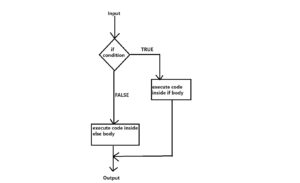# Mastering Python if-elif-else loop [Step by Step Guide]## What is loop in Python ?

In this tutorial, we will learn about mastering Python if-elif-else loop .In general loop means, doing something again and again unless some condition is satisfied. This is exactly what we do in Python as well. Loop provides us a way to executed a piece of code again and again until a condition is met. For example, let’s say you have 3 cars and 2 bikes. For each of your vehicle, you want to check if it is a car or bike. You can use if-else loop here. It will let you know which vehicle is car and which vehicle is bike. We will understand this in more practical way by writing some cool code in the upcoming section.

## Why loop is really needed ?

Like in any other programming language, loop is very important feature in Python as well. It comes handy when you have to do some repetitive tasks and perform some action without writing the same code again and again. There are primarily three types of loop in Python and then there are sub-types of loops as well. They are:

• if loop
• for loop
• while loop

This tutorial is dedicated for if loop and it’s sub-types (i.e if-else, if-elif-else and nested if loop). We will cover each of the sub-types of if loop in more detail in the upcoming sections with example code.

## Mastering Python if-elif-else loop [Step by Step Guide]

Now that we know why the loop really exist in programming world and what these are, let’s understand the flow of if-else loop using a block diagram that is shown below. What it does is that, it reads a user input. The user input is first checked against the condition defined in if block. If the user input satisfy the if condition and turns out to be true, the body inside the if will get executes. If user input does not satisfy the if condition, code inside the else block will be executed. This flow has been shown in below block diagram.### Understand if loop

The best way to understand any concept is by visualizing it and we are going to do exactly the  same to understand end to end flow of all if-else use cases going forward. Let us write a simple piece of Python code what uses if condition as shown below.

```age = 10
if age >= 20:

name = "linuxnasa"
if name == "linuxnasa":
print(f"You are {name}")```
In the above code, we have defined a variable age and assigned it a value 10. In the if condition, age value will be checked. If age value is greater than or equal to 20, it will execute the print statement inside if block. Next, we have declared and defined another variable name. We have assigned the value linuxnasa to it. If name is equal to the string linuxnasa, the print statement inside second if block will be executed.

OUTPUT

`You are linuxnasa`

### Understand if-else loop

We are now familiar with if working. One thing we must notice in the above code is that if if condition is not met, it does not really do anything. But we want to do something, atleast print some message saying no condition is met so that user knows the code inside if block is not executed. We can do that by adding else code block as shown below.

```age = 10
if age >= 20:

else:
print("Age is below 20")

name = "linuxnasa"
if name == "linuxnasa":
print(f"You are {name}")

else:
print("You are Unknown")```

OUTPUT

```Age is below 20
You are linuxnasa```

### Understand nested if-else loop

It’s all good so far. We have if-else to handle both satisfactory and unsatisfactory condition. But what if we want add multiple conditions to the if statement, can we really do that? . Well, the answer is yes, in Python, we use nested if blocks to add more than one condition based on use cases. Let us see the example below.

```age = 30
if age > 0:
if age >= 20:

OUTPUT

`Your age is 30 years`

In the above example code, the first if condition checks if the age variable value is greater than zero. If the condition executed is true, it will move to next if block (called nested if block) and check the next if condition. If the condition executed in second if block is true, it will execute the print statement and returns the message on console.

### Understand if-elif-else loop

We have something called elif which comes with if loop. what it really does is similar to if . We add a condition to elif block. this condition will be checked only if if condition is executed as false. If both if and elif condition is executed as false, the code inside the else body will get executed. I have implemented the concept in the below code.

```age = -2
if age < 0:
print("Age can not be less than zero")

elif age >= 20:

else:
print("Age is less than 20")```

OUTPUT

```Age can not be less than zero
```

## Summary

if loop is more powerful than we can think of in Python. No matter how easy or complex code you write, if will always comes if picture to save your life. So make sure you get your hand dirty as much as you can with if loop. You can also refer to Python’s official documentation at python.org for more understanding.